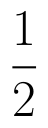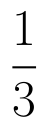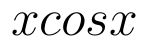Select Page

# 12 Science CBSE Maths Solutions for MCQ Probability in English

12 Science CBSE Maths Solutions for MCQ Probability in English to enable students to get Solutions in a narrative video format for the specific question.

Expert Teacher provides 12 Science CBSE Maths Solutions for MCQ Probability through Video Solutions in English language. This video solution will be useful for students to understand how to write an answer in exam in order to score more marks. This teacher uses a narrative style for a question from Probability not only to explain the proper method of answering question, but deriving right answer too.

Please find the question below and view the Solution in a narrative video format.

Question:

Solution Video in English:

You can select video Solutions from other languages also. Please check Solutions in ( Hindi )

## Similar Questions from CBSE, 12th Science, Maths, Probability

Question 1 : Assume that the chances of a patient having a heart attack are 40%. Assume that a meditation and yoga course reduces the risk of heart attack by 30% and the prescription of certain drugs and certain restrictions reduces the risk by 25%. At a time, a patient choses only one of the two options with equal probabilities. After going through one of the two options, the patient is selected at random who is suffering from a heart attack. Find the probability that the patient followed a course of meditation and yoga. Write the value referred here.      (View Answer Video)

Question 2 : Consider the experiment of tossing a coin. If the coin shows head, toss is done again, but if it shows tail, then throw a die. Find the conditional probability of the events that ' the die shows a number greater than 4', given that ' there is atleast one tail'.    (View Answer Video)

Question 3 :  Probability of solving specific problem independently by A and B areandrespectively. If both try to solve the problem, independently, then find the probability that exactly one of them solves the problem.  (View Answer Video)

Question 4 : A coin is biased so that the heads is 2 times as likely to occur as tail. If the coin is tossed twice, find the probability distribution of number of tails. Hence find the mean of the distribution.  (View Answer Video)

Question 5 : Ifandthen find(View Answer Video)

### Application of Integrals

Question 1 : Area lying in the first quadrant and bounded by the circleand the lines x =0 and x = 2 is (View Answer Video)

Question 2 : Using integration, find the area of the triangle formed by a positive x-axis and tangent and normal to the circleat. (View Answer Video)

Question 3 : Find the area bounded by the curveand the line x = 4y - 2. (View Answer Video)

Question 4 :

Find the area of the given curves and given lines:and x-axis

Question 5 : Find the area bounded by curves. (View Answer Video)

### Application of Derivatives

Question 1 : Find the maximum profit that a company can make if the profit function is.(View Answer Video)

Question 2 : The line y = x + 1 is a tangent to the curveat the point: (View Answer Video)

Question 3 : The function f is a differentiable function and satisfies the functional equation f(x) + f(y) = f(x + y) – xy – 1for every pair x, y of real numbers. If f(1) = 1, then the number of integers n ≠ 1 for which f(n) = n is_______________. (View Answer Video)

Question 4 : Ifthen the approximate value of f(3.02) is : (View Answer Video)

Question 5 : A cylindrical tank of radius 10 m is being filled with wheat at the rate of 314 cubic meters per hour. Then the depth of the wheat is increasing at the rate of: (View Answer Video)

### Continuity and Differentiability

Question 1 :  Find the second order derivative of the function. (View Answer Video)

Question 2 :  Differentiate w.r.t.x the function. (View Answer Video)

Question 3 : Findfor the function. (View Answer Video)

Question 4 : Differentiate the functionwith respect to x. (View Answer Video)

Question 5 : Differentiate the functionwith respect to x. (View Answer Video)